Next: How many facets can Up: Convex Polyhedron Previous: How do we measure   Contents

## How many facets does the average polytope with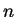vertices in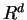have?

Clearly we need to define a probability distribution of points to answer the question.

Perhaps the most interesting describution for which the answer is known is the uniform distribution on the unit sphere. The results of Buchta et al [BMT85] show that the expected number of facets is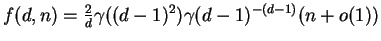assymtotically with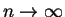. The important fact is that it depends linearly onessentially. Here the functionis defined recursively by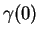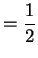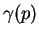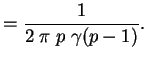Just to see how large the slope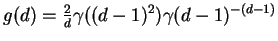of this linear'' function inis, we calculate it for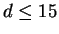: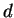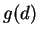2 1 3 2 4 6.76773 5 31.7778 6 186.738 7 1296.45 8 10261.8 9 90424.6 10 872190. 11 9.09402E+06 12 1.01518E+08 13 1.20414E+09 14 1.50832E+10 15 1.98520E+11Next: How many facets can Up: Convex Polyhedron Previous: How do we measure   Contents
Komei Fukuda 2004-08-26